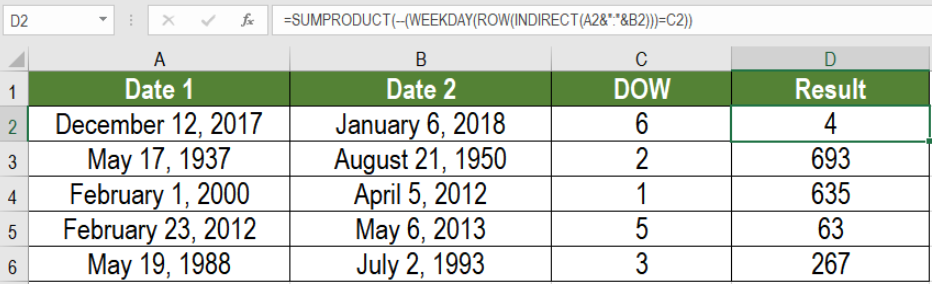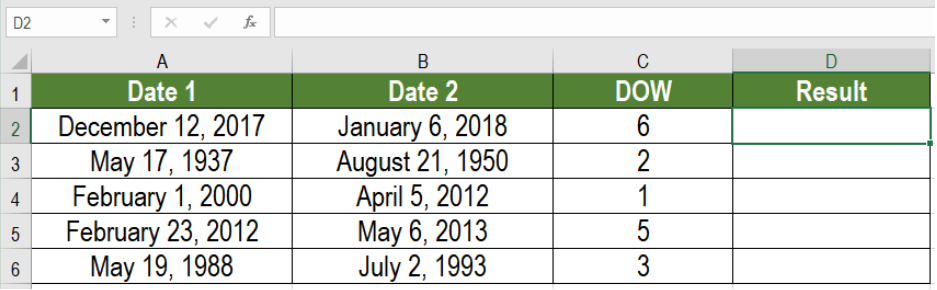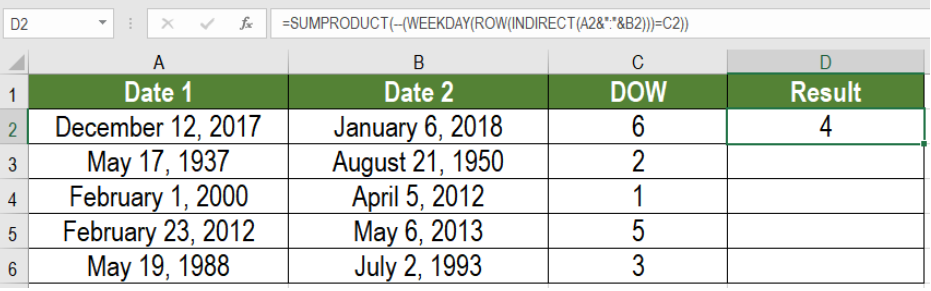Get instant live expert help with Excel or Google Sheets“My Excelchat expert helped me in less than 20 minutes, saving me what would have been 5 hours of work!”

#### Post your problem and you’ll get expert help in seconds.

Your message must be at least 40 characters
Our professional experts are available now. Your privacy is guaranteed.

# Count day of week between dates

We can count day of week between dates in Microsoft Excel. Doing it manually can be very frustrating. We can extract the results automatically. The functions used to count days of week between dates are – ROW, WEEKEND, INDIRECT and SUMPRODUCT. In this tutorial, we will learn how to count day of week between dates in Excel.Figure 1. Example of How to Count Day of Week Between Dates

Excel can easily count the day of week between dates. For example, if we have two dates,  January 1, 2019, and January 28, 2019. We can find out how many Saturday exists between these two dates.

## Generic Formula

`=SUMPRODUCT(--(WEEKDAY(ROW(INDIRECT(date1&":"&date2)))=dow))`

## How this formula works

The main purpose of this function is to extract the number of time a day comes between two certain dates. The formula consists of four functions. Here, WEEKDAY searches if the number of mentioned dates fall on the certain day of the week. Excel abbreviates this day of the week as DOW. This formula gives a number which is between one to seven indicating a certain day from the week. Excel counts the days of the week as-

1- Sunday,

2- Monday

3- Tuesday

4- Wednesday

5- Thursday

6- Friday

7-Saturday

Excel works with dates as serial numbers starting from Jan 1, 1900.  The INDIRECT and ROW functions work together to create an array of numbers from the dates. The INDIRECT function helps to interpret the concatenated numbers to count as rows. The ROW function returns an array consisting these numbers. These numbers are converted to dates with the WEEKDAY function. Finally, SUMPRODUCT processes these dates and returns the count.

## Setting up Data

The following example consists some sample dates. Column A and B have these dates. Column C contains the day of the week represented as numbers.Figure 2. Setting up the Data

To count the day of week in column D, we need to:

• Go to cell D2.
• Assign the formula `=SUMPRODUCT(--(WEEKDAY(ROW(INDIRECT(A2&":"&B2)))=C2)) `to D2.
• Press Enter to apply the formula to D2.
• Drag the formula from cells D2 to D6 to copy the formula to the entire column.Figure 3. Applying the Formula to the Data

This will show the day of week between dates in column D. For example, cell D2 will get display the result 4. This means there are four Fridays between 12th December, 2017 and 6th January,2018.

Most of the time, the problem you will need to solve will be more complex than a simple application of a formula or function. If you want to save hours of research and frustration, try our live Excelchat service! Our Excel Experts are available 24/7 to answer any Excel question you may have. We guarantee a connection within 30 seconds and a customized solution within 20 minutes.

### Did this post not answer your question? Get a solution from connecting with the expert.Another blog reader asked this question today on Excelchat:
Solution examplesin range b2:o2, I am trying to write a formula to count if the values are equal to 1 thru 27.
Solved by E. L. in 52 minsI have a google sheet that takes information from a google form, that creates a row every time a form is submitted. Since it is a new row that is created, rather than filling in the next row down, the formulas I have applied to some of the columns do not work. The same is true when I try and link the data to another sheet to bypass this problem. This is probably quite a general functionality issue so I won't send the actual document but let me know if you need it
Solved by F. F. in 13 minsi have a table with Dates, First names , Last names and i want to count how many times a name occurs, but if a name occurs more than once on a particular date i need to only count it once. this is on excel and not google sheets
Solved by F. H. in 31 minsI need cell B2 Green if cells G2:Q2 are empty. If cells G2:Q2 have any text in any cell then i need B2 to be red.
Solved by C. W. in 20 minsHi, I need a formula to work out how many values are less than a certain time value, simple "countif" do not work as it is a filtered spreadsheet. Here's the formula that I have got so far: SUMPRODUCT(SUBTOTAL(3,OFFSET(Current_Month!N\$2:N\$10000,ROW(Current_Month!N\$2:N\$10000)-MIN(ROW(Current_Month!N\$2:N\$10000)),,1)),--(Current_Month!N\$2:N\$10000>"24:0:0")
Solved by K. L. in 30 mins## Subscribe to Excelchat.coAnother blog reader asked this question today on Excelchat: